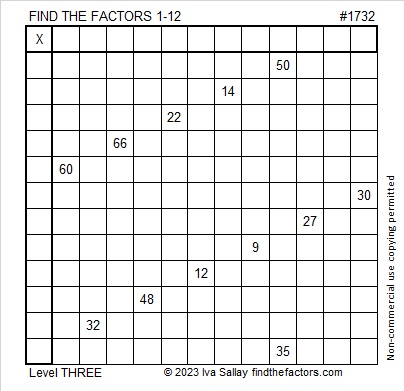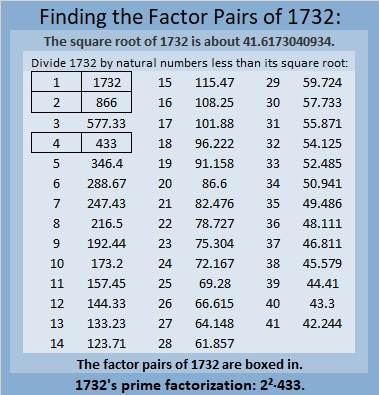# 1732 Is a Thousand Times More Than…

Contents

### Today’s Puzzle:

If you know the common factor of 50 and 35 that will put only numbers from 1 to 12 in the first column and the top row of this multiplication table puzzle, then you will have completed the first step in solving the puzzle. Afterward, just work from the top of the puzzle to the bottom filling in factors as you go. That’s how you solve these level-three puzzles.### Factors of 1732:

• 1732 is a composite number.
• Prime factorization: 1732 = 2 × 2 × 433, which can be written 1732 = 2² × 433.
• 1732 has at least one exponent greater than 1 in its prime factorization so √1732 can be simplified. Taking the factor pair from the factor pair table below with the largest square number factor, we get √1732 = (√4)(√433) = 2√433.
• The exponents in the prime factorization are 2 and 1. Adding one to each exponent and multiplying we get (2 + 1)(1 + 1) = 3 × 2 = 6. Therefore 1732 has exactly 6 factors.
• The factors of 1732 are outlined with their factor pair partners in the graphic below.### More About the Number 1732:

1732 is the sum of two squares:
34² + 24² = 1732.

1732 is the hypotenuse of a Pythagorean triple:
580-1632-1732, calculated from 34² – 24², 2(34)(24), 34² + 24².
It is also 4 times (145-408-433).

1732 is a palindrome in base 15 because
7(15²) + 10(15) + 7(1) = 1732.

I remember one of my college professors telling his class that
√2 is about 1.4, and Valentine’s day is February 14,
√3 is about 1.7, and Saint Patrick’s day is March 17.

That’s how I remember those values, but this tweet reminded me that 1732 is a thousand times more than the square root of three rounded to three decimal places. It also makes a reference to the square root of two.

This site uses Akismet to reduce spam. Learn how your comment data is processed.